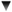ArticleS. UncleBob.

### Safe Computations

My last blog about Convex Hulls involved an interesting topic; the safety of using math in a computer. The Jarvis March algorithm involves calculating the polar angle of each point from a reference point. Radians would be perfect except that it is computationally expensive to calculate the radian angle. (It involves inverse trig functions). However, as several of the convex hull articles point out, you don't actually need the angle; the tangent of the angle will do. This is true because as the angle increases so does the tangent. Or rather, if a > b then tan a > tan b. (So long as you stay within a quadrant). Since the only thing the Jarvis March does with an angle is to compare it to other angles, the tangent will do. This is good news because tangents are very easy to calculate: dy/dx.

The problem with tangents is that they range from -infinity to +infinity. You can detect the infinite case easily enough (dx == 0) but you still have to deal with numbers that can get very large. In a Convex Hull algorithm the chances that dx between two points will be small enough to explode the result is not insignificant. Besides, checking for the infinity case is a pain.

The argument could be made that the tangent (slope) is safe for this particular algorithm since the tangent can't really get any larger than dx can be small, so there can't really be any loss of precision. But I don't like it anyway. Dealing with functions that can roar off to infinity is inherently risky. So I decided to see if there might be a better solution.

I came up with the idea of mapping the angle to the perimeter of a square whose vertices were on the axes.
`        |   *p        *  /      * | @    *   |/  *--*-----O-----*----    *   |   *      * | *        *        |`

In the diagram above, the angle of the line OP from vertical is related to the permeter of the square from the topmost point of the square to the intersection (@) of OP with the square. How can we calculate this perimeter?

If we restrict our math to the first quadrant, then a little math shows that the square of the perimeter, D^2 = 2/(m+1)^2 where m is the slope of OP. What we want to do is get rid of m since it can roar off to infinity if OP gets close to vertical.

`So: D^2 = 2/((m+1)^2)    D   = sqrt(2)/(m+1)        = sqrt(2)/(1+(dy/dx))        = sqrt(2)/((dy+dx)/dx)        = sqrt(2)*dx/(dy+dx)`

Now since all we are after is a function that increases as the angle increases, the sqrt(2) is meaningless. So dx/(dy+dx) is good enough. Better yet this function has a range of [0,1] which keeps the floating point exponent very close to zero. The check for dx==0 is no longer necessary since the function yeilds zero if dx is zero and 1 if dy is zero, and is only undefined if the two points are coincident (which we can check for in a different way).

This is nice. The function is fast and well behaved. No infinities to worry about.

But was it really necessary, or should I have have just used m?

!commentForm -rSun, 10 Sep 2006 02:05:36, xreborner,
Only using cross multiplication to compare two points is enough. Is it very fast (only multiplication operations, no divisions, sqrt or even trigonometric functions) and simple.Thu, 7 Sep 2006 22:06:17, Ravi Venkataraman,
It was not stated in the original article that the square intersected the axes at (0,1), (1,0), (0,-1) and (-1,0)

That assumes a square of side sqrt(2) which was unstated. In my earlier comment, I did refer to some unstated assumptions.

The use of the term "perimeter" is confusing, too. The perimeter of a 2-D figure is the sum of the lengths of its sides. In your example, the perimeter is simly 4 * sqrt(2). Here, D is the distance from the point (0,1) to the intersection of the line with the square. It is definitely not the perimeter of the square.

In order to get rid of infinities when dealing with angles and their trigonometric ratios/values, it may turn out to be simpler to consider either the sine or cosine of an angle, since these are always between 0 and 1. The sine is also increasing in the same interval that the tangent increases (and decreases with the tangent).Thu, 7 Sep 2006 17:24:35, Uncle Bob, D^2 = 2/(m+1)^2 correct?
The point @ can be found by solving the two equations:
 y=mx where m is the slope of O@ y=-x+1 e.g. The line from (0,1) to (1,0)

This give us @x=1/(m+1) and @y=m/(m+1)
Applying the distance formula from (0,1) to (@x,@y) gives us D^2 = 2/(m+1)^2

Did I make a mistake?Thu, 7 Sep 2006 08:22:30, Ravi Venkataraman, I don't get it
I do not see how the formula D^2 = 2/(m+1)^2 can be correct. On the left hand side we have a quantity that is the square of a length, while on the right hand side we have a dimensionless quantity (tangent of an angle is a ratio of the lengths of two sides, hence a real number and dimensionless). How can the formula hold? For the same reason, the formula for D in terms of dx and dy seems wrong.

I guess that some other assumption must have been made, such as the length of the line O@ or the length of the side of the square.